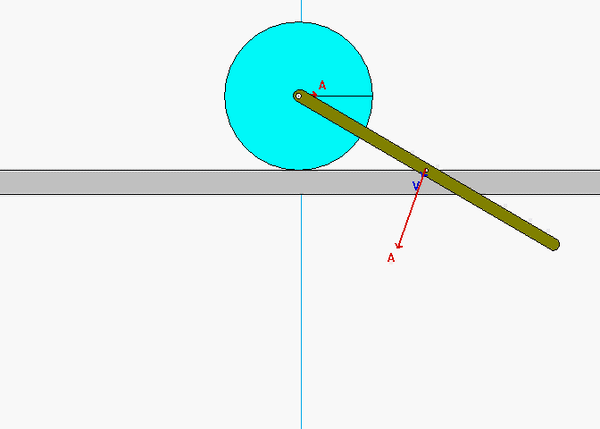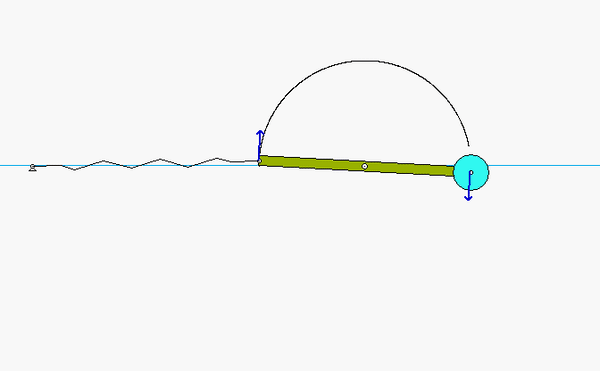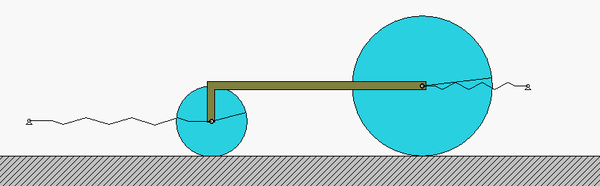Samples of oscillatory motion

Shown below are samples of problems that we have considered (or will consider) this semester that exhibit oscillatory motion. For problems that we have considered earlier, we solved for measurements of the motion such as velocity or acceleration at a single instant in time. In our study of vibrations in the course, we will consider a subset of such problems (those having linear equations of motion) for which we can readily solve for displacement vs. time for all instances in time. This requires us to solve differential equations rather than algebraic equations to find the answers.